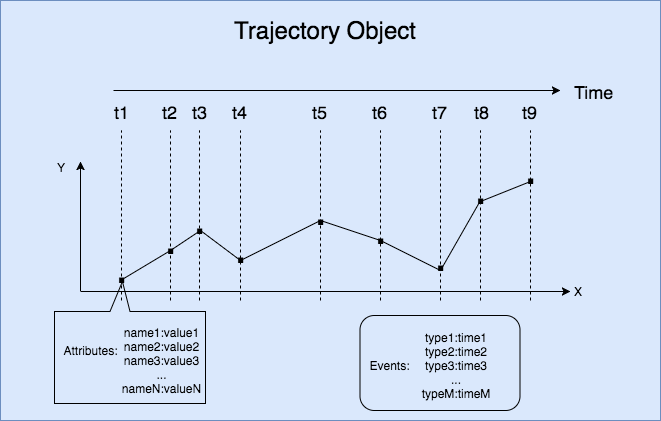This topic introduces the basic concepts of Trajectory SQL.

## Trajectory objects

Concept Description
Trajectory Point The spatio-temporal object that consists of the spatial location and attributes of a moving object at a specific point in time. The spatial location can be represented by 2D or 3D coordinates. The attributes can be a number of fields that use different data types.
Trajectory Object The high-dimensional object that consists of trajectory points and events. Specifically, the high-dimensional object includes time, space, attributes, and events.
Trajectory Timeline The time value sequence of a trajectory. The time values in the sequence must be consecutive.
Trajectory Spatial The spatial geometry of a trajectory. In most cases, the spatial geometry is described by using the LineString data type.
Trajectory Leaf The spatial location of a moving object at a specific point in time. The spatial location is a trajectory point.
Trajectory Attributes The attributes that describe the trajectory of a moving object at different trajectory points. These attributes include velocity and direction.
Trajectory Attribute Field The attribute field that describes the trajectory of a moving object. For example, these attribute fields include the velocity field. The number of values for an attribute field is the same as the number of trajectory points that is owned by the moving object.
Trajectory Field Value The value of an attribute field at a specific point in time.
Trajectory Events The events that occur along a trajectory. For example, these events include the refueling, breakdown, and car lock events of a moving car along the trajectory. An event consists of the event type ID and the event time.

The following figure shows the trajectory of a moving object.## BoxNDF objects

Trajectory-related objects (trajectories and geometries) can be complex. Therefore, cubes that are easier to calculate than these trajectory-related objects may be used to describe queries or simplify computing operations. The BoxNDF data type is used to describe cubes.

A BoxNDF object is a multi-dimensional spatio-temporal cube that is expressed by the minimum and maximum values on the following four coordinate axes: x, y, z, and t. The z axis indicates space, and the t axis indicates time. Different cubes may include different axes. For example, some cubes include only the x and y axes, and some include all of the x, y, z, and t axes.

When you run a query, you can use a cube to specify the query scope. In addition, you can use a bounding box of the trajectory or geometry type to make the query easier. The following figure shows the trajectory and bounding box of an object that moves on the x, y, and t axes.